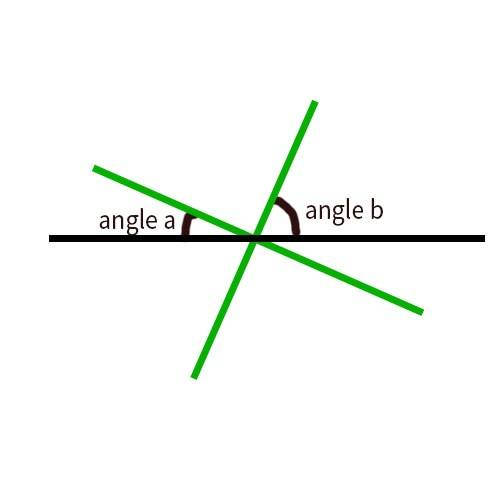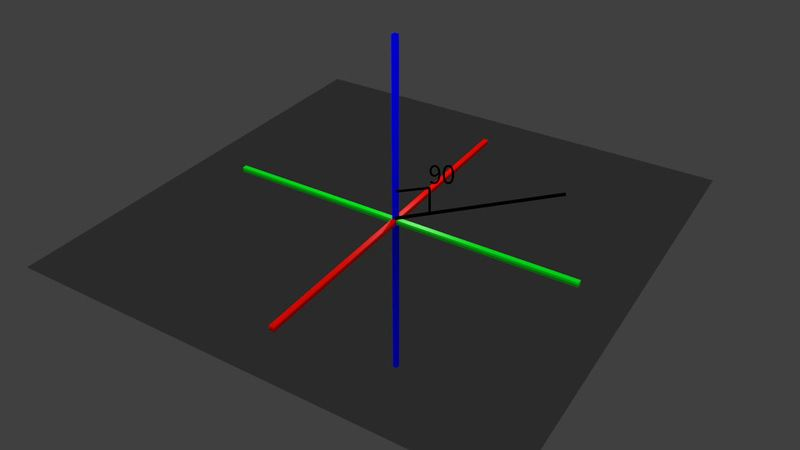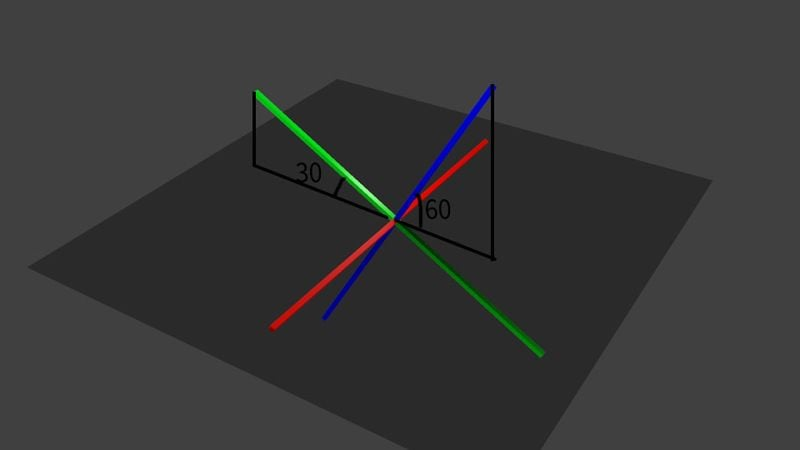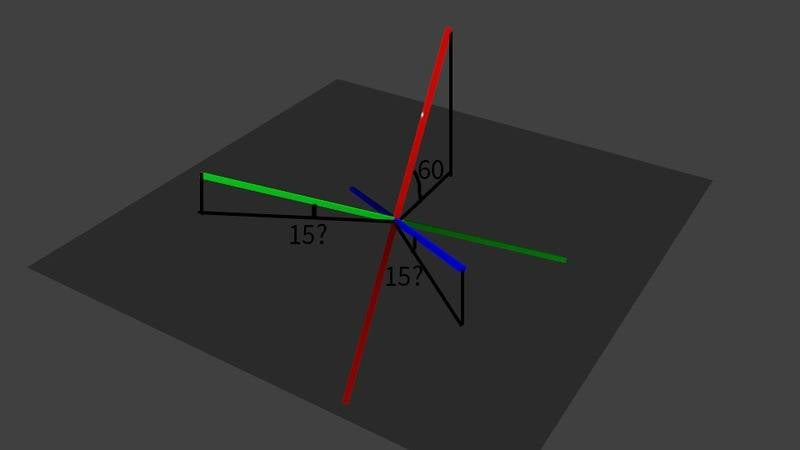# The sum of angles in 3D is not 90 while in 2D it is?

• aosome23

#### aosome23

Sorry for the confusing tittle but I could not explain it better. Here is what I am trying to ask:

When you have 2 axis(x and y) such as the image below, the sum of the two angles, a and b will always be equal to 90 degrees.
a + b = 90degreesHowever when you add a 3rd axis(x, y and z, making it 3D), the total sum seems to differ depending on the angles of each axis. I have tried it multiple times and the largest angles seems to be around 106 degrees. The sum of the angles starts to get bigger as the angles of each axis becomes closer to each other.

i tested this out in a game engine called unity so my programming might be wrong but I highly doubt that since it is only a couple lines of code. If anyone understands how to code in unity, here is the code:

float angleX = Vector3.Angle(transform.right, new Vector3(transform.right.x, 0.0f, transform.right.z));
float angleY = Vector3.Angle(transform.up, new Vector3(transform.up.x, 0.0f, transform.up.z));
float angleZ = Vector3.Angle(transform.forward, new Vector3(transform.forward.x, 0.0f, transform.forward.z));

When the 3 angles are added up together it ranges from 90 degrees to about 106 degrees as I mentioned above. So, why does this happen? Is this supposed to happen? Or am I doing something wrong?

Last edited:
Sorry for the confusing tittle but I could not explain it better. Here is what I am trying to ask:

When you have 2 axis(x and y) such as the image below, the sum of the two angles, a and b will always be equal to 90 degrees.
a + b = 90degreesHowever when you add a 3rd axis(x, y and z, making it 3D), the total sum seems to differ depending on the angles of each axis. I have tried it multiple times and the largest angles seems to be around 106 degrees. The sum of the angles starts to get bigger as the angles of each axis becomes closer to each other.

i tested this out in a game engine called unity so my programming might be wrong but I highly doubt that since it is only a couple lines of code. If anyone understands how to code in unity, here is the code:

float angleX = Vector3.Angle(transform.right, new Vector3(transform.right.x, 0.0f, transform.right.z));
float angleY = Vector3.Angle(transform.up, new Vector3(transform.up.x, 0.0f, transform.up.z));
float angleZ = Vector3.Angle(transform.forward, new Vector3(transform.forward.x, 0.0f, transform.forward.z));

When the 3 angles are added up together it ranges from 90 degrees to about 106 degrees as I mentioned above. So, why does this happen? Is this supposed to happen? Or am I doing something wrong?
I have no idea what you're asking. Your code is not enlightening, since it's not clear what the code is supposed to be doing.

Going back to your drawing, are you assuming that the two green lines are perpendicular? If so, then the angles a and b will add to 90°. However, if the two green lines aren't perpendicular, then a and b don't add to 90°.

aosome23 said:
However when you add a 3rd axis(x, y and z, making it 3D), the total sum seems to differ depending on the angles of each axis.
The total sum of what? What three angles are you adding? A picture of what you're trying to figure out would be helpful.

I have no idea what you're asking. Your code is not enlightening, since it's not clear what the code is supposed to be doing.

Going back to your drawing, are you assuming that the two green lines are perpendicular? If so, then the angles a and b will add to 90°. However, if the two green lines aren't perpendicular, then a and b don't add to 90°.

The total sum of what? What three angles are you adding? A picture of what you're trying to figure out would be helpful.

Sorry for the confusion... Long story short, I wanted to know if the same rule for 2 perpendicular lines applies for 3 perpendicular lines.
For 2 perpendicular lines(x and y):
angle between the first green line and the black line + angle between the second green line and the black line = 90 degrees no matter what

so I was wondering if there was 3 perpendicular lines(x y and z) does the same rule apply?
angle between the first green line and the black line + angle between the second green line and the black line + angle between the third green line and the black line = 90 degrees?

To answer this question. You have to look at geometries that remove the controversial parallel postulate of euclidean geometry.

To narrow the search down. Look at spherical trigonometry.

Sorry for the confusion... Long story short, I wanted to know if the same rule for 2 perpendicular lines applies for 3 perpendicular lines.
For 2 perpendicular lines(x and y):
angle between the first green line and the black line + angle between the second green line and the black line = 90 degrees no matter what

so I was wondering if there was 3 perpendicular lines(x y and z) does the same rule apply?
angle between the first green line and the black line + angle between the second green line and the black line + angle between the third green line and the black line = 90 degrees?
Isn't this last condition always wrong if the black line is in the direction of one of the other lines? The sum should be 180 degrees then.

Sorry for the confusion... Long story short, I wanted to know if the same rule for 2 perpendicular lines applies for 3 perpendicular lines.
For 2 perpendicular lines(x and y):
angle between the first green line and the black line + angle between the second green line and the black line = 90 degrees no matter what

so I was wondering if there was 3 perpendicular lines(x y and z) does the same rule apply?
This is still not clear. What is needed is fewer words and more drawings.
aosome23 said:
angle between the first green line and the black line + angle between the second green line and the black line + angle between the third green line and the black line = 90 degrees?0(red axis)+0(green axis)+90(blue axis) =900+30+60 = 90

So these two are rotated only on the red axis, which means that it is only 2 dimensional. So when you introduce rotation on other axis will the sum of these angle remain 90 degrees such on the image below?You should be able to solve this problem with a general roll-pitch-yaw rotation matrix and calculation of the angles between the rotated x,y,z-unit vectors and the plane shown in your picture.

You should be able to solve this problem with a general roll-pitch-yaw rotation matrix and calculation of the angles between the rotated x,y,z-unit vectors and the plane shown in your picture.
Thank you for the reply but I do not know how to do the matrix calculations and such..
Would you mind doing an example?

I would love to try it later maybe! But now I have to go to bed! If somone else wants to try it, feel free.

Notice that the general matrix (according to wikipedia)

##
\begin{pmatrix}

\cos \Theta \cos \Psi &
\sin \Phi \sin \Theta \cos \Psi - \cos \Phi \sin \Psi &
\cos \Phi \sin \Theta \cos \Psi + \sin \Phi \sin \Psi\\

\cos \Theta \sin \Psi &
\sin \Phi \sin \Theta \sin \Psi + \cos \Phi \cos \Psi &
\cos \Phi \sin \Theta \sin \Psi - \sin \Phi \cos \Psi \\

-\sin \Theta &
\sin \Phi \cos \Theta &
\cos \Phi \cos \Theta

\end{pmatrix}
##

reduces to some special cases if some of the angles ##\Phi, \Theta, \Psi## are zero.

Lets first check out the cases where we have only rotation around one axis. The sum of the angles should be 90 degrees in this case.

##\Theta=0, \Psi=0## yields
##
\begin{pmatrix}
1 &
0 &
0 \\

0 &
\cos \Phi &
- \sin \Phi \\

0 &
\sin \Phi &
\cos \Phi
\end{pmatrix}
##

which is an ordinary rotation matrix around a single axis.

The rotated unit vectors (the colums of the matrix) are

##e'_x = \begin{pmatrix} 1\\0\\0\end{pmatrix}##.
##e'_y = \begin{pmatrix} 0\\ \cos\Phi \\ \sin\Phi\end{pmatrix}##
##e'_z = \begin{pmatrix} 0\\ -\sin\Phi \\ \cos\Phi\end{pmatrix}##

Since we are interested in the angles in respect to the x-y-plane we know that the sine of the angles must be just the z-component (the last component) of the rotated unit vectors.

Therefore ##\sin\varphi_x=0##, ##\sin\varphi_y=\sin\Phi##, ##\sin\varphi_z=\cos\Phi=\sin(90^\circ-\Phi)##. Therefore ##\varphi_x=0##, ##\varphi_y=\Phi## and ##\varphi_z=90^\circ-\Phi##. Adding them together yields ##\varphi_x+\varphi_y+\varphi_z = 90^\circ##.

This was for rotation about one axis. If someone else wants to try rotation about two axes, feel free.

Ok, so much about taking a nap!

Just looking at the general matrix above shows that even or the general case (rotation about three axes) the rotated unit vectors are

##e'_x = \begin{pmatrix} \dots\\\dots\\ -\sin \Theta\end{pmatrix}##.
##e'_y = \begin{pmatrix} \dots\\ \dots \\ \sin \Phi \cos \Theta \end{pmatrix}##
##e'_z = \begin{pmatrix} \dots\\ \dots\\ \cos \Phi \cos \Theta\end{pmatrix}##

With the same argumentation as above we have now

##\sin\varphi_x=-\sin\Theta##,
##\sin\varphi_y=\sin\Phi\cos\Theta##,
##\sin\varphi_z=\cos\Phi\cos\Theta##.

Lets use some identities to see if the sum of the angles can be bigger than ##90^\circ##.

##\cos \Theta \cos \Phi = {{\cos(\Theta - \Phi) + \cos(\Theta + \Phi)} \over 2}##.
##\sin \Theta \cos \Phi = {{\sin(\Theta + \Phi) + \sin(\Theta - \Phi)} \over 2}##.

Since all angles are positive,
##\varphi_x=\Theta##,
##\varphi_y=\arcsin(\sin\Phi\cos(\Theta))=\arcsin({{\cos(\Theta - \Phi) + \cos(\Theta + \Phi)} \over 2})##
##\varphi_z=\arcsin(\cos(\Phi)\cos(\Theta))=\arcsin( {{\sin(\Theta + \Phi) + \sin(\Theta - \Phi)} \over 2})##

Now let's just choose ##\Theta=\Phi=45^\circ## to get

##\varphi_x=45^\circ##
##\varphi_y=\arcsin(\sin\Phi\cos(\Theta))=\arcsin({{\cos(0) + \cos(90^\circ)} \over 2})=\arcsin(\frac{1}{2})=30^\circ##
##\varphi_z=\arcsin(\cos(\Phi)\cos(\Theta))=\arcsin( {{\sin(90^\circ) + \sin(0)} \over 2})=\arcsin(\frac{1}{2})=30^\circ##

Summation: ##\varphi_x+\varphi_y+\varphi_z=105^\circ \neq 90^\circ##- Seems to fit with your observation.

Now, for extra credit, one could ask for which rotation angles the sum reaches its maximum.

Ok, so much about taking a nap!

Just looking at the general matrix above shows that even or the general case (rotation about three axes) the rotated unit vectors are

##e'_x = \begin{pmatrix} \dots\\\dots\\ -\sin \Theta\end{pmatrix}##.
##e'_y = \begin{pmatrix} \dots\\ \dots \\ \sin \Phi \cos \Theta \end{pmatrix}##
##e'_z = \begin{pmatrix} \dots\\ \dots\\ \cos \Phi \cos \Theta\end{pmatrix}##

With the same argumentation as above we have now

##\sin\varphi_x=-\sin\Theta##,
##\sin\varphi_y=\sin\Phi\cos\Theta##,
##\sin\varphi_z=\cos\Phi\cos\Theta##.

Lets use some identities to see if the sum of the angles can be bigger than ##90^\circ##.

##\cos \Theta \cos \Phi = {{\cos(\Theta - \Phi) + \cos(\Theta + \Phi)} \over 2}##.
##\sin \Theta \cos \Phi = {{\sin(\Theta + \Phi) + \sin(\Theta - \Phi)} \over 2}##.

Since all angles are positive,
##\varphi_x=\Theta##,
##\varphi_y=\arcsin(\sin\Phi\cos(\Theta))=\arcsin({{\cos(\Theta - \Phi) + \cos(\Theta + \Phi)} \over 2})##
##\varphi_z=\arcsin(\cos(\Phi)\cos(\Theta))=\arcsin( {{\sin(\Theta + \Phi) + \sin(\Theta - \Phi)} \over 2})##

Now let's just choose ##\Theta=\Phi=45^\circ## to get

##\varphi_x=45^\circ##
##\varphi_y=\arcsin(\sin\Phi\cos(\Theta))=\arcsin({{\cos(0) + \cos(90^\circ)} \over 2})=\arcsin(\frac{1}{2})=30^\circ##
##\varphi_z=\arcsin(\cos(\Phi)\cos(\Theta))=\arcsin( {{\sin(90^\circ) + \sin(0)} \over 2})=\arcsin(\frac{1}{2})=30^\circ##

Summation: ##\varphi_x+\varphi_y+\varphi_z=105^\circ \neq 90^\circ##- Seems to fit with your observation.

Now, for extra credit, one could ask for which rotation angles the sum reaches its maximum.

Okay thank you! So i guess my guess was pretty off :'(...

So the reason I was asking if the sum of these angles was 90 degrees is because I wanted to express these angles in percentages.
If only one axis is rotated, the sum is always 90 degrees so all I have to do is the angle*100/90 and I would get the percentage. However this does not work when all axis are rotated. It seems like the maximum sum of the angles are arcsin(3^-1/2)*3 or about 105.793 degrees.

When more than one axis is rotated, the denominator has to be a number between 105.793 and 90 degrees. And I don't think this method will work.

Are there any alternatives?

Although it may seem pointless, I really want to be able to show these angles in percentages to calculated the weighted average of the angles

Thank you :D
Also thank you DarthMatter for the solvings... It seems that I need to study a lot more math

Well, if it seems pointless, why do it? I guess you could just divide by 105.793 degrees for a simple solution. If you want a more sophisticated solution, you could calculate ##\varphi_x + \varphi_y + \varphi_z## and divide the single angles by it. I am not sure what that number means geometrically though.

Also thank you DarthMatter for the solvings... It seems that I need to study a lot more math
Studying maths never is a bad idea, but this may seem more complicated than it is. The idea behind the rotation matrices is that you know that you can write each vector ##\vec{v}## as ##\vec{v}=x \begin{pmatrix} 1 \\ 0 \\ 0 \end{pmatrix}+y \begin{pmatrix} 0 \\ 1 \\ 0 \end{pmatrix}+z \begin{pmatrix} 0 \\ 0 \\ 1 \end{pmatrix}##. So instead of rotating a general vector all at once, you can just rotate ##\begin{pmatrix} 1 \\ 0 \\ 0 \end{pmatrix}##, ##\begin{pmatrix} 0 \\ 1 \\ 0 \end{pmatrix}## and ##\begin{pmatrix} 0 \\ 0 \\ 1 \end{pmatrix}##. The picture for this is rotating all axes, but letting the components fixed. This will result in a rotated vector. Call the rotated unit vectors ##\vec{e}_x'##, ##\vec{e}_y'##, ##\vec{e}_z'##. Then your rotated vector will simply be ##\vec{v}'=x\vec{e}_x'+y\vec{e}_y'+z\vec{e}_z'##. This is the reason why the rotation matrix consists of the rotated unit vectors.